# 530 and Level 1

The first three perfect numbers are 6, 28, and 496. Their sum is 530.

Can you find the greatest common factors for each of these Pythagorean triples?

• 46-528-530
• 192-494-530
• 280-450-530
• 318-424-530Print the puzzles or type the solution on this excel file: 10 Factors 2015-06-22

—————————————————————————————————

• 530 is a composite number.
• Prime factorization: 530 = 2 x 5 x 53
• The exponents in the prime factorization are 1, 1, and 1. Adding one to each and multiplying we get (1 + 1)(1 + 1)(1 + 1) = 2 x 2 x 2 = 8. Therefore 530 has exactly 8 factors.
• Factors of 530: 1, 2, 5, 10, 53, 106, 265, 530
• Factor pairs: 530 = 1 x 530, 2 x 265, 5 x 106, or 10 x 53
• 530 has no square factors that allow its square root to be simplified. √530 ≈ 23.02172886644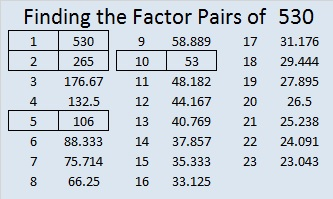—————————————————————————————————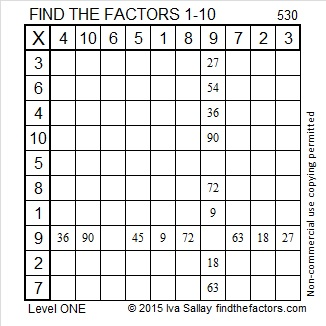# 528 and Level 6

528 feet is one tenth of a mile. Joseph Nebus has written a fascinating post on WHY a mile is 5280 feet. Factoring that number is part of the reason.

Since 528 = (32 x 33)/ 2, we know that 528 is the 32nd triangular number.

Thus, 1 + 2 + 3 + 4 + . . . . . + 29 + 30 + 31 + 32 = 528.

528 can be written as the difference of two squares six different ways: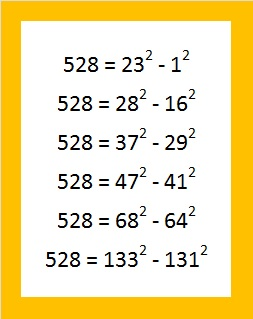How did I find them? Choose a factor pair where both factors are even or both factors are odd. Average the two factors and that will be the first number to be squared. Square it and subtract 528. Take the square root of the result. That number is the second number in the difference of two squares.Print the puzzles or type the solution on this excel file: 12 Factors 2015-06-15

—————————————————————————————————

• 528 is a composite number.
• Prime factorization: 528 = 2 x 2 x 2 x 2 x 3 x 11, which can be written 528 = (2^4) x 3 x 11
• The exponents in the prime factorization are 4, 1 and 1. Adding one to each and multiplying we get (4 + 1)(1 + 1)(1 + 1) = 5 x 2 x 2 = 20. Therefore 528 has exactly 20 factors.
• Factors of 528: 1, 2, 3, 4, 6, 8, 11, 12, 16, 22, 24, 33, 44, 48, 66, 88, 132, 176, 264, 528
• Factor pairs: 528 = 1 x 528, 2 x 264, 3 x 176, 4 x 132, 6 x 88, 8 x 66, 11 x 48, 12 x 44, 16 x 33 or 22 x 24
• Taking the factor pair with the largest square number factor, we get √528 = (√16)(√33) = 4√33 ≈ 22.97825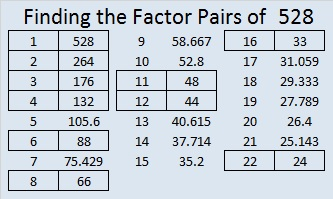—————————————————————————————————# 527 and Level 5

527 is the sum of consecutive primes including all the prime numbers in its prime factorization. Can you list those consecutive primes?Print the puzzles or type the solution on this excel file: 12 Factors 2015-06-15

—————————————————————————————————

• 527 is a composite number.
• Prime factorization: 527 = 17 x 31
• The exponents in the prime factorization are 1 and 1. Adding one to each and multiplying we get (1 + 1)(1 + 1) = 2 x 2 = 4. Therefore 527 has exactly 4 factors.
• Factors of 527: 1, 17, 31, 527
• Factor pairs: 527 = 1 x 527 or 17 x 31
• 527 has no square factors that allow its square root to be simplified. √527 ≈ 22.95648—————————————————————————————————# 519 and Level 4

519 = 167 + 173 + 179, three consecutive primes. The difference between each of those prime numbers is 6 so one of them is also one of the factors of 519. The other factor that pairs up with it should be obvious, too.

519 is the hypotenuse of the Pythagorean triple 156-495-519. Can you find the greatest common factor of those three numbers?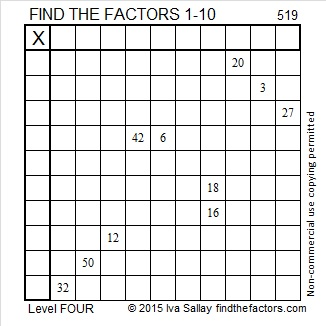Print the puzzles or type the solution on this excel file: 10 Factors 2015-06-08

—————————————————————————————————

• 519 is a composite number.
• Prime factorization: 519 = 3 x 173
• The exponents in the prime factorization are 1 and 1. Adding one to each and multiplying we get (1 + 1)(1 + 1) = 2 x 2 = 4. Therefore 519 has exactly 4 factors.
• Factors of 519: 1, 3, 173, 519
• Factor pairs: 519 = 1 x 519 or 3 x 173
• 519 has no square factors that allow its square root to be simplified. √519 ≈ 22.781571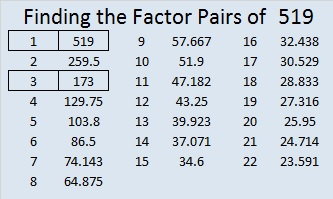—————————————————————————————————# 463 and Level 5

463 is the sum of consecutive primes, too! Check the comments to see if any of my readers finds out what those consecutive primes are.

This Level 5 puzzle might be a little harder than usual. If you’ve solved a Level 5 puzzle before, see if you can meet this challenge!Print the puzzles or type the solution on this excel file:  10 Factors 2015-04-13

—————————————————————————————————

• 463 is a prime number.
• Prime factorization: 463 is prime.
• The exponent of prime number 463 is 1. Adding 1 to that exponent we get (1 + 1) = 2. Therefore 463 has exactly 2 factors.
• Factors of 463: 1, 463
• Factor pairs: 463 = 1 x 463
• 463 has no square factors that allow its square root to be simplified. √463 ≈ 21.5174How do we know that 463 is a prime number? If 463 were not a prime number, then it would be divisible by at least one prime number less than or equal to √463 ≈ 21.5174. Since 463 cannot be divided evenly by 2, 3, 5, 7, 11, 13, 17, or 19, we know that 463 is a prime number.

—————————————————————————————————# 442 and Level 1

442 is the sum of two square numbers two different ways: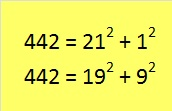Even number 442 is also the sum of consecutive primes. Will the number of consecutive primes be even or odd? If you figure out what those prime numbers are, look in the comments to see if you were right.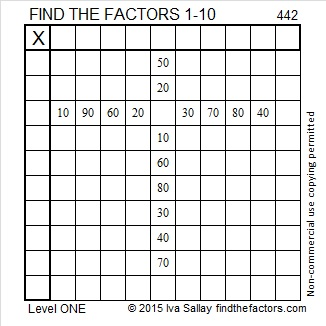Print the puzzles or type the factors on this excel file: 10 Factors 2015-03-30

Here is the factoring information for 442:

• 442 is a composite number.
• Prime factorization: 442 = 2 x 13 x 17
• The exponents in the prime factorization are 1, 1, and 1. Adding one to each and multiplying we get (1 + 1)(1 + 1)(1 + 1) = 2 x 2 x 2 = 8. Therefore 442 has exactly 8 factors.
• Factors of 442: 1, 2, 13, 17, 26, 34, 221, 442
• Factor pairs: 442 = 1 x 442, 2 x 221, 13 x 34, or 17 x 26
• 442 has no square factors that allow its square root to be simplified. √442 ≈ 21.0238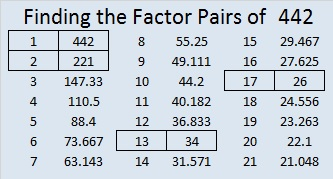442 is the hypotenuse of four Pythagorean triples:

• 170-408-442 which is [5-12-13] times 34
• 208-390-442 which is [8-15-17] times 26
• 280-342-442 which is [140-171-221] times 2
• 42-440-442 which is [21-220-221] times 2